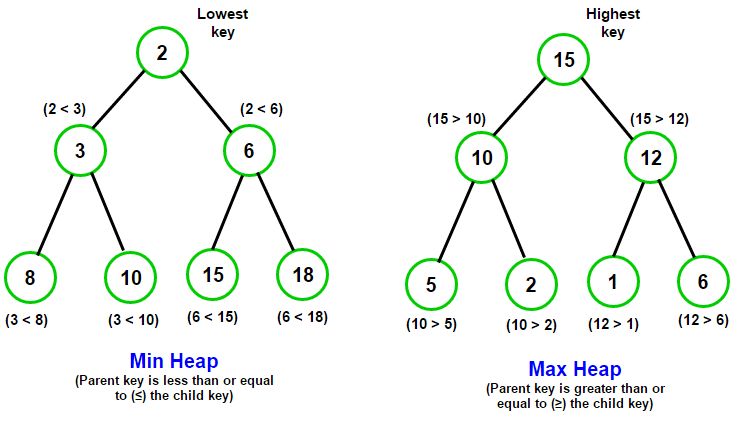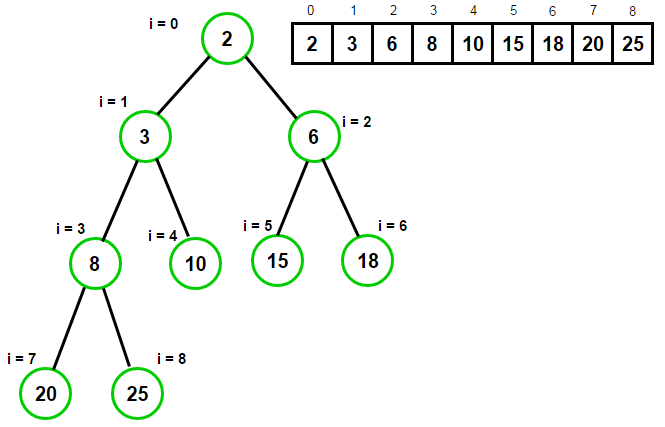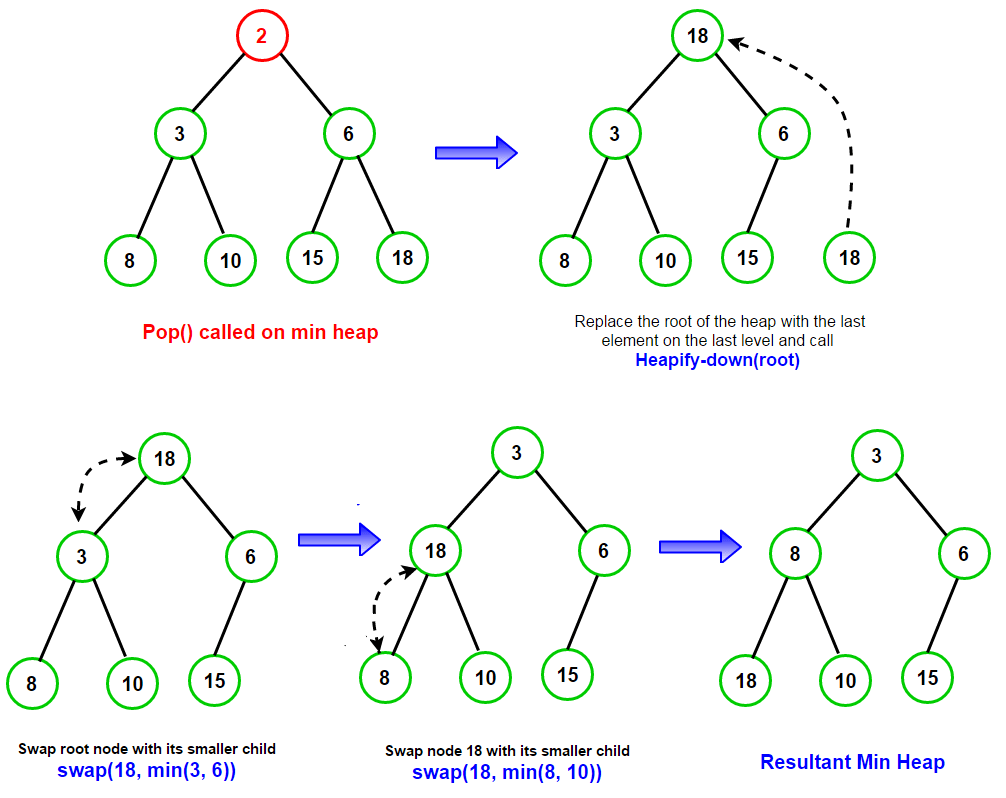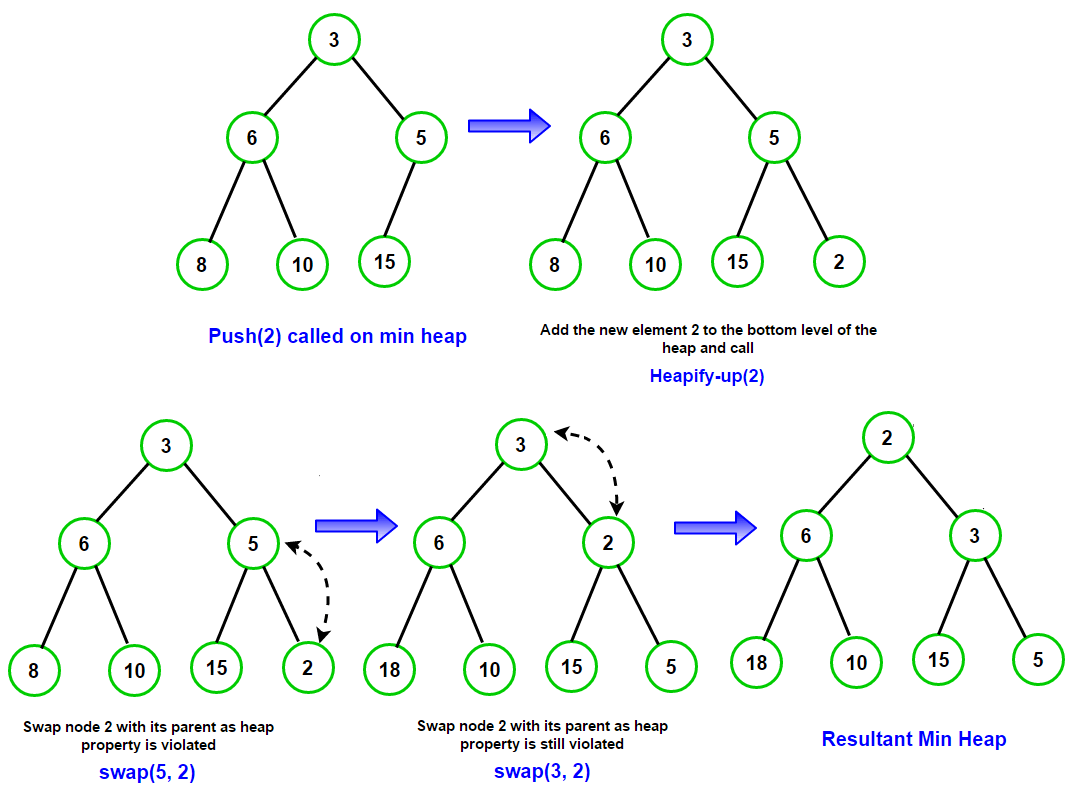# Introduction to Priority Queues using Binary Heaps

In this article, we will introduce a very important data structure Priority Queues and discuss how we can implement them using (Binary) Heaps.

## Priority Queue

A priority queue is an ADT (Abstract Data Type) for maintaining a set S of elements, with each element has a “priority” associated with it. In a priority queue, an element with high priority is served before an element with low priority or vice versa. If two elements have the same priority, they are served according to their order in the queue. It basically supports the following operations –

1. `push(x):` insert element x in the set S – usually a O(log(n)) operation

2. `top()` or `peek():` returns the element of S with highest (or lowest) priority (but do not modify the queue) – O(1) operation

3. `pop():` returns and removes the element of S with highest (or lowest)  priority – usually a O(log(n)) operation

## Heap Data Structure

Heap data structure can be used to implement priority queue. A heap data structure should not be confused with the heap which is a pool of memory used for dynamically memory allocation. A common implementation of a heap is the binary heap, which is defined as a binary tree with two additional properties –

• Structural property: A binary heap is a complete binary tree i.e. all levels of the tree, except possibly the last one (deepest) are fully filled, and, if the last level of the tree is not complete, the nodes of that level are filled from left to right.

• Heap Property: The key stored in each node is either “greater than or equal to” or “less than or equal to” the keys in the node’s children.

## Min Heap & Max Heap

A heap can be classified further as either a “max heap” or a “min heap”.

• In a max heap, the keys of parent nodes are always greater than or equal to those of the children and the highest key is in the root node.

• In a min heap, the keys of parent nodes are less than or equal to those of the children and the lowest key is in the root node.The highest (or lowest) priority element is always stored at the root in binary heap. Binary heap have smallest possible height of log N where N is the number of nodes in the heap.

## Heap operations

A binary heap is a complete binary tree but we usually never use binary tree for implementing heaps. We store keys in an array and using their relative positions within that array to represent child-parent relationships. Below diagram shows an array representing min-heap.The complete binary tree maps the binary tree structure into the array indices where each array index represents a node. The index of the node’s parent, left or right child are simple expressions. For a zero-based array, the root node is stored at index 0. If i is the index of the current node, then

PARENT(i) = floor((i-1)/2)
LEFT-CHILD(i) = 2*i + 1
RIGHT_CHILD(i) = 2*i + 2

Min Heap Property says – A[PARENT[i]] <= A[i]
Max Heap Property says – A[PARENT[i]] >= A[i]

### Heapify operation –

Heapify operation forms the crux of all major Heap operations. It can be implemented as heapify-up and heapify-down.

`Heapify-down(i)` can be invoked if element A[i] violates the heap property with its two direct children. `Heapify-down(i)` converts the binary tree rooted at index i to a heap by moving A[i] down the tree. It does so by comparing A[i] with its left and right child and swapping A[i] with the smaller child for min-heaps & the larger child for max-heaps and then calling Heapify-down on the corresponding child i.e. Heapify-down(LEFT_CHILD(i)) or Heapify-down(RIGHT_CHILD(i)). The process is repeated till heap property is fixed. The complexity of heapify-down operation is O(log(n)).

Heapify-down is used in `pop()` operation of the binary heap. The idea is to replace the root of the heap with the last element on the last level and call Heapify-down on the root. The following diagram illustrates the process –`Heapify-up(i)` can be invoked if parent of an element A[i] violates the heap property. `Heapify-up(i)` converts the binary tree into a heap by moving A[i] up the tree. It does so by comparing A[i] with its parent and swapping the two if heap property is violated. We then call Heapify-up on the parent i.e. Heapify-up(PARENT(i)). The process is repeated till heap property is fixed. The complexity of heapify-up operation is O(log(n)).

Heapify-up is used in push() operation of the binary heap. The idea is to add the new element to the bottom level of the heap and call Heapify-up on the last node. The following diagram illustrates the process –## Heap Applications –

Heaps are crucial in several efficient graph algorithms such as Dijkstra’s shortest path algorithm and Prim’s algorithm for minimum spanning tree. They are also used in the heapsort sorting algorithm and in Huffman coding (A Data Compression technique).

References –(1 votes, average: 5.00 out of 5)Loading...